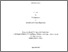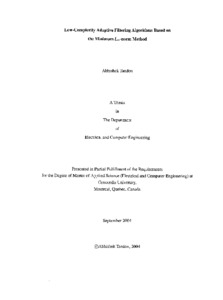Title:

# Low-complexity adaptive filtering algorithms based on the minimum L [infinity]-norm method

Tandon, Abhishek (2004) Low-complexity adaptive filtering algorithms based on the minimum L [infinity]-norm method. Masters thesis, Concordia University.Preview
Text (application/pdf)
MQ94711.pdf - Accepted Version
2MB

## Abstract

The complexity of an adaptive filtering algorithm is proportional to the tap length of the filter and hence, may become computationally prohibitive for applications requiring a long filter tap. In this thesis, we provide a framework for developing low-complexity adaptive filter algorithms by utilizing the concept of partial-updating along with the technique of finding the gradient vector in the hyperplane based on the L {592} -norm criterion. The resulting algorithm should have low-complexity not only because of the updating of only a subset of the filter coefficients at each time step, but also from the fact that updating a filter coefficient using the algorithm based on L {592} -norm requires less number of operations compared to the L 2 -norm algorithm. Two specific coefficient selection techniques, namely the sequential and M -Max coefficient selection techniques, are considered in this thesis. Statistical analyses of these two algorithms are carried out to derive the evolution equations for the mean and mean-square of the filter coefficient misalignment as well as to obtain stability bounds on the step-size of the two algorithms. Further, these analyses are used to show that the algorithm employing the M -Max coefficient selection technique can achieve a convergence rate that is closest to the full update algorithm. As a consequence, even though there are various ways of selecting a subset of the filter coefficients, the study of the other techniques becomes redundant. Simulations are carried out to validate the results obtained from the statistical analyses of the algorithms. The concept of developing algorithms based on the partial-updating and L {592} -norm is extended to proportionate adaptive filtering. Finally, the performance of the proposed adaptive filtering algorithms as well as that of the existing ones is studied in echo cancellation.

Divisions: Concordia University > Gina Cody School of Engineering and Computer Science > Electrical and Computer Engineering Thesis (Masters) Tandon, Abhishek xiv, 84 leaves : ill. (some col.) ; 29 cm. Concordia University M.A. Sc. Electrical and Computer Engineering 2004 Swany, M. N. S and Ahmad, M. O TK 7872 F5T26 2004 8098 Concordia University Library 18 Aug 2011 18:15 13 Jul 2020 20:03 https://concordiauniversity.on.worldcat....
All items in Spectrum are protected by copyright, with all rights reserved. The use of items is governed by Spectrum's terms of access.

Repository Staff Only: item control pageResearch related to the current document (at the CORE website)
Back to top